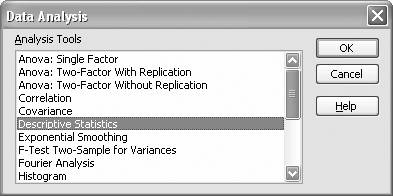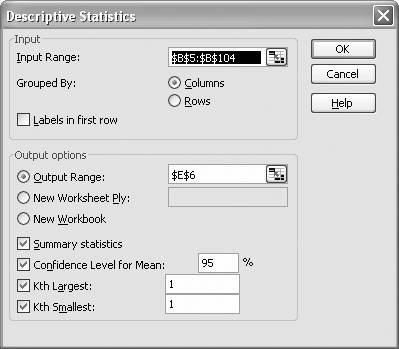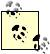# Computing Summary Statistics

Problem

You need to compute summary statistics (measures of central tendency and dispersion) but are not sure what support Excel offers for these calculations.

Solution

Excel offers many built-in spreadsheet functions allowing you to quickly compute summary statistics. Further, the Analysis ToolPak offers a Descriptive Statistics tool . You can perform the same statistical calculations offered in the Descriptive Statistics tool using spreadsheet functions. There are, however, caveats that you need to know about. Read the following discussion for more information.

Discussion

I'll show you how to perform basic statistical calculations in Excel on an example set of data. The dataset I'll use was downloaded from the National Institute of Standards and Technology statistical reference dataset page at http://www.itl.nist.gov/div898/strd/general/dataarchive.html. The dataset consists of speed of light measurements from a study conducted by Michelson in 1879. The data consists of 100 values representing the speed of light in units of millions of meters per second. Figure 5-1 illustrates a portion of this data along with several computed statistics for the dataset.

The dataset is in column B, labeled Speed of Light (millions of m/s). There are 100 values in this column, but only the first dozen and a half or so are shown in the figure.

Column F contains statistics for this dataset computed using the Analysis ToolPak's Descriptive Statistics tool. The corresponding labels contained in column E indicate what each value represents. Column H contains statistics computed using Excel's built-in spreadsheet formulas. I'll show you how to use both methods.

First, to use the Descriptive Statistics tool, you have to open the Analysis ToolPak by selecting Tools [Image_Link]/images/U2192.jpg border=0> Data Analysis from the main menu bar. This action opens the Data Analysis dialog shown in Figure 5-2.

Select Descriptive Statistics from the list of available tools and press OK to open the Descriptive Statistics dialog box shown in Figure 5-3.

In the Input Range field, type (or select directly from your spreadsheet) the cell range containing the data you want to analyze. Since the example data is in a column, I selected the Grouped By: Columns option.

For this example, I decided to place the output on the same spreadsheet, next to the dataset. Therefore, I specified cell E6 for the Output Range. This specified cell represents the upper-left cell of the output table. You may choose to place the output on a new worksheet, in which case you need to specify a worksheet name.

Figure 5-1. Summary statistics of speed of light measurementsFigure 5-2. Data Analysis dialog boxI checked all of the options shown in the lower part of the dialog box in Figure 5-3 in order to generate all the available statistics. Pressing OK generates the results shown earlier in Figure 5-1.

Figure 5-3. Descriptive Statistics dialog boxYou can compute the same statistics using only Excel's built-in functions. Cells H8 through H23 in Figure 5-1 contain the same set of statistics, computed using Excel's functions. Table 5-1 shows the cell formula I used to compute each statistic.

Table 5-1. Summary statistics cell formulas

Statistic

Cell formula

Mean

=AVERAGE(Data)

Standard Error

=H12/SQRT(H20)

Median

=MEDIAN(Data)

Mode

=MODE(Data)

Standard Deviation

=STDEV(Data)

Sample Variance

=VAR(Data)

Kurtosis

=KURT(Data)

Skewness

=SKEW(Data)

Range

=h18-H17

Minimum

=MIN(Data)

Maximum

=MAX(Data)

Sum

=SUM(Data)

Count

=COUNT(Data)

Largest(1)

=LARGE(Data,1)

Smallest(1)

=SMALL(Data,1)

Confidence Level(95.0%)

=CONFIDENCE(0.05,H12,100)

Most of these formulas require the cell range containing the input data as an argument. For convenience, I named the cell range B5 to B104 (containing the input data) Data. (See Recipe 1.14 to learn how to name cells and cell ranges.)

Table 5-1 illustrates that Excel has built-in formulas for all of these basic statistics with two exceptions. There's no formula for computing the Standard Error (standard error of the mean in this case). Nor is there a formula for computing the Range. However these two statistics are easily computed using the results of other formulas. For example, to compute the range you simply take the difference between the Maximum and the Minimum. To compute the Standard Error of the mean, you simply divide the Standard Deviation by the square root of Count.

If you take a close look at Figure 5-1, you'll see that the statistics computed using Excel's formulas exactly match those computed with the Analysis ToolPak with one exception: the values for the 95% confidence interval are different. This difference is due to the fact that the CONFIDENCE function uses the inverse of a standard normal distribution to compute the confidence interval, whereas the Analysis ToolPak uses the inverse of Student's t-distribution. Excel does provide other functions that allow you to compute confidence intervals using Student's t-distribution. See Recipes 5.3 and 8.8 for more information.

I should also mention that some of Excel's statistics functions (including those in the Analysis ToolPak) have historically dealt poorly with some troublesome datasets. For example, if your dataset contained very large numbers with very small differences between them, the Descriptive Statistics tool would compute the standard deviation and variance incorrectly, and this would then affect results of other statistics that used those values. The workaround in this case is to center your data by subtracting the mean from each value (or by shifting the data by a sufficiently large value), and then compute the standard deviation and variance based on the centered data.

In Excel 2003, it appears that the statistics functions have been fixed, so this error no longer occurs. To see if this error exists on your version of Excel, enter the following data in a spreadsheet and compute the standard deviation: 10000000001, 10000000002, 10000000003, 10000000004, and 10000000005. The average, standard deviation, and variance for this dataset are 10000000003, 1.58113883, and 2.5, respectively. In older versions of Excel, the standard deviation and variance would come out to 0.

If you center your data by subtracting the mean from each value, your dataset should look like this: -2, -1, 0, 1, 2. The standard deviation and variance should be the same as for the uncentered dataset. In older versions of Excel, the standard deviation and variance for this centered dataset should come out correctly.

If you shift the original dataset by subtracting, say, 1e10 from each value, you end up with a dataset that looks like this: 1, 2, 3, 4, 5. The standard deviation and variance should be the same as in the previous two cases. Again, older versions of Excel deal with this shifted dataset just fine and compute the correct statistics.This sort of troublesome data can also adversely affect the results of statistical tests covered later in this chapter. Here again, you should center the data before conducting such tests.

Excel's help guide includes a complete list of all its built-in statistical functions. You can find this list by searching Excel's help for the topic "Statistical Functions."

Excel also has several built-in summation functions that are very handy for statistical calculations that involve sums, sums of squares, sums of squared differences, and so on. These functions are discussed in Recipe 7.1.

Moreover, Excel has built-in functions to support regression and curve fitting. These, along with other regression techniques, are discussed in Chapter 8.Excel Scientific and Engineering Cookbook (Cookbooks (OReilly))
ISBN: 0596008791
EAN: 2147483647
Year: N/A
Pages: 206
Authors: David M Bourg